### Performing a Chain Analysis in Excel

Chain Analysis is the name I’ve given to the act of finding sequential, potentially related phone calls in phone records. The job is to identify if a particular number called a second number and if that second number called a third number within a specific time frame, say 10 minutes. I call these sequences of calls a chain and it can be used to identify whether someone might be issuing orders or instructions via third parties. The analysis can be done in Excel and while it involves some moderately-complicated Excel functions, once the spreadsheet is set up, subsequent analyses can be done easily.

Getting Started

To start, the first thing you need to do is arrange your data properly. In the screen shot below I have arranged some sample data where column A lists the calling number, column B lists the called number and column C lists the date and time of the call. In order for this technique to work properly it is important that the data set be sorted on the date time column.

For simplicity sake (and ease of reading) I’ve replaced the phone numbers with letters but really any string will work in the analysis. Likewise, I only have 7 rows of data but you can have a whole bunch more and the analysis will work just fine.

While we’ll need a few extra columns for functions there is no reason you can’t keep additional data in the rest of the columns. As long as you sort everything properly the rest of your information will tag along as you perform the analysis.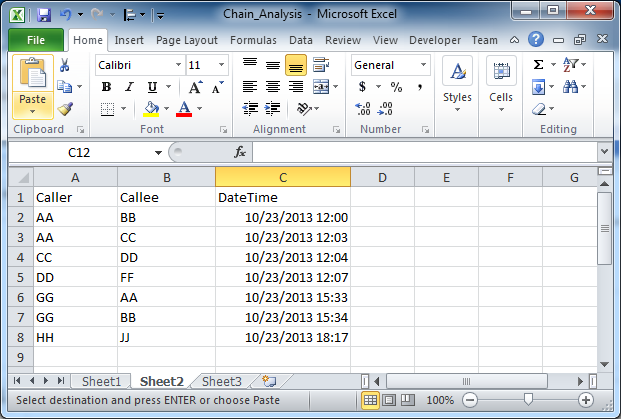Notice in the list that there are some chains that are easily identified without resorting to a function. Notice how, on row 3, AA calls CC and then CC calls DD a minute later. Similarly, on row 6, GG calls AA and then a minute later GG calls BB. This is also a chain to be identified. The trick now is to write a function that is able to flag these rows so that we don’t have to rely on picking them out manually.

To flag the row we’re first going to create a new column that, for each row, “looks ahead” at the rows coming up. What we want the function to do is look ahead to see how many subsequent rows fall within the time frame window we’re interested in. In the discussion above I mentioned 10 minutes so, for each row, we want to count how many subsequent rows occur within 10 minutes of the date and time of the current row. The screen shot below captures the function that I wrote for cell D2.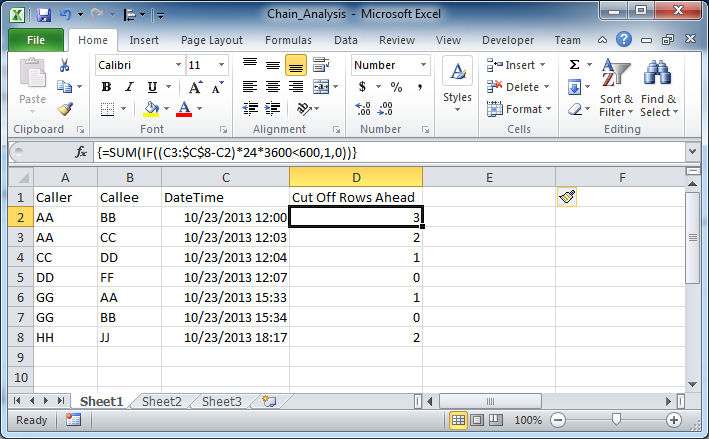The very first thing to notice is that the function is wrapped in curly braces like this: {<function>}. This means that the function is what’s known as an array formula rather than a traditional scalar formula. To enter an array formula you click in the cell like you normally would but instead of hitting enter when you’re done you need to hit ctrl-shift-enter (at the same time) to tell Excel that it should be considered an array formula.

This particular function needs to be used as an array formula because we are asking the function to compare each subsequent cell to the current one in the IF statement, so we’re comparing an array of values rather than just a single one. Also, when you’re typing the function into the cell, don’t include the curly braces, Excel will add those after when you correctly use ctrl-shift-enter to complete the array function.

Let’s examine the IF statement next. The inner most part of the function is a request to check and see if the difference between cell C3 and cell C2 is less than 600 (our 5 minute cut-off). Since the date and time values in the C column are in Excel time we need to convert them to seconds before we do the comparison, which is why I multiply them by 24 (hours) and then 3600 (seconds) to get the difference into seconds. In the IF statement, if the difference between C3 and C2 is less than 600, then we return a 1 and if not we return a 0. Now, the tricky part is to wrap your head around the idea that, because this is an array function, we can use the expression C3:\$C\$8-C2 to do all of the comparisons at the same time. This means that we are doing C3-C2, C4-C2, C5-C2, etc, all the way to C8-C2. For each one of them we do the x24 x3600 conversion and comparison against 600 seconds. For each one of them we are getting either a 1 or a 0. The power of the array function method is that we can do all of those calculations at the same time, in the same cell.

Have a look at the SUM function that wraps the IF statement. Knowing that this IF statement is multivalued makes the SUM function make more sense. The role of the SUM is now to sum up all of the 1s that were returned by each of the IF statements. Have a look at the value of D2, it’s 3. It’s 3 because the date/time in C3, C4 and C5, are both within 10 minutes (600 seconds) of the value in C2.

Array formulas can be filled down just like regular functions so I have filled down the rest of the rows with this function. The key thing to remember is that you only need to compare the current row to the all the subsequent rows, and so the important bit of the function, the C3:\$C\$8-C2 bit, will always have the current row in the C2 position and the next row in the C3 position. As an example, in cell D4 the function is =SUM(IF((C5:\$C\$8-C4)*24*3600<600,1,0)) . Note that for row 4, C4 is used to subtract and the range of cells to check starts at C5.

Figuring Out the Look Ahead Row Number

The next step in the analysis is create a new column to hold the end row value for the look ahead. If the values in column D tell us how many rows to look ahead then the values in column E tell us what row that actually is. Have a look at the function in cell E2 in the screen grab below.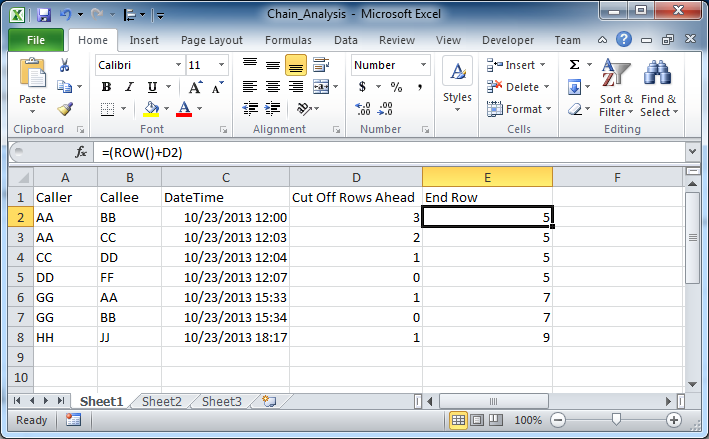This function uses the ROW() function to first tell us what row we are currently in. Then the value of D2 is added to that to arrive at the final value. For cell E2 the value is 5 since the ROW() function returns 2 and the value of D2 is 3. What this means is that we only need to look ahead to row 5, from row 2, to find all the phone calls that occurred within the 10 minute window. Anything beyond row 5 is more than 10 minutes after the call in row 2. The end row is an important component of the next part of the analysis as we need to be able to specify exactly what cell range we should be looking at for determining chains.

Flagging the Chained Calls

Have a look at the screen grab for the final step of the analysis, the step where each row is flagged as part of a chain or not.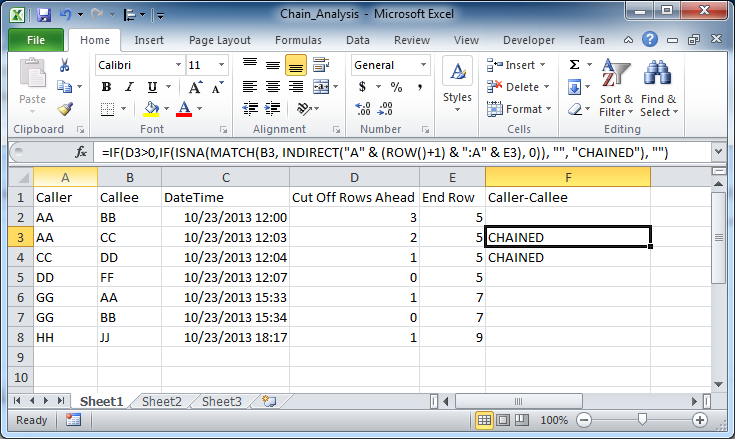The heart of the function is the use of the MATCH function to compare the value in column B, the Callee column, with the values in the Caller column for all the rows up to the end row identified in column E. In order to do that we need to use the INDIRECT function, which is used here to assemble a cell range using the ROW() function and the value from column E. Inside the INDIRECT is (“A” & (ROW()+1) & “:A” & E3). If you evaluate this we can see that ROW()+1 becomes 4 (since ROW() is 3 and 3+1 is 4) and the value in E3 is 5, which leaves (“A” & 4 & “:A” & 5). If you slam all that together (which is what the &s are for) you end up with A4:A5, which is the range of cells we want to test our MATCH against.

Something else you need to know: when MATCH can’t find a match it returns NA, which is why the entire MATCH call is wrapped in an ISNA function call. If MATCH returns an NA value, then ISNA will return true, otherwise it returns false. I then feed that true or false into an IF statement (which is only interested in evaluating things to true or false anyway). If the value in the IF is true, that is, MATCH returned NA, then IF prints nothing, “”, to the cell. However, if MATCH returns a value and therefore ISNA returns false, then the IF statement will print “CHAINED” in the cell. For good measure, I wrap the whole thing in another IF statement to check and see if the look ahead rows in cell D3 are greater than 0. If not, there is no way there can be a chain, so the function just prints the empty string “” and we skip the whole MATCH, ISNA, IF process.

So let’s have a look at the results the function spit out. We can see that two chains have been identified. The first is on row 3 where AA called CC and then CC called DD within 10 minutes. This is what the CHAINED in cell F3 is identifying. The second CHAINED is for the chain that begins when CC calls DD and then DD calls FF (on row 5) within 10 minutes. The take away here is that chains are identified on the row in which they begin so it’s up to you to follow the chains from the starting point where they are identified.

Bonus Chains

The above example looks at Caller-Callee chains but almost the same function can be used to flag Caller-Caller chains, the ones where a caller calls a number and then within the time interval the same caller calls another number. In the screen shot below Caller-Caller chains have been identified for the second row and the sixth row and the only difference between the functions in column F and column G is that the MATCH in the column G version is being done on the values in column A (e.g. A2) rather than column B (e.g. B2). All the same logic applies but the change in the matching column changes the chaining subject.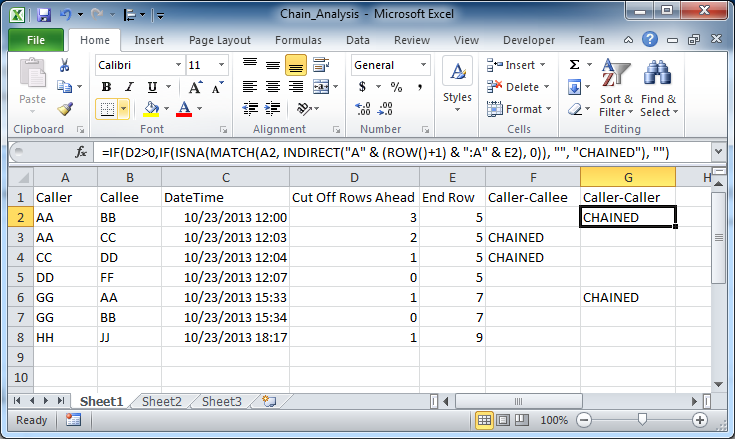As can be seen in the chart, row 2 is a Caller-Caller chain because AA calls BB and then within 10 minutes AA calls CC. Similarly, on row 6, GG calls AA then GG calls BB with 10 minutes. Just like with the Caller-Callee chains above, the CHAINED flag is listed on the row where the chain begins and it’s up to the analyst to follow the chain through.

Conclusion

While the functions in this post can seem a little hairy at the start I think, with a little exploration, that you can become comfortable enough with them to trust the results. While it’s true that a custom VBA function could be written to perform this analysis I think it’s important to find solutions that first attempt to use the built-in Excel functions before resorting to custom code. I hope this post has demonstrated how a fairly sophisticated kind of analysis can be performed with just the tools that come in standard Excel. Good luck!